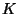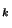SPIN GEOMETRY AND THE DIRAC OPERATOR

Blaine Lawson

Syllabus - Spring, 2002

Part I. The algebraic theory of spinors.

(i) Clifford algebras: structure and representation theory.

(ii) Spin groups and spinor representations.

Part II. K-Theory and characteristic classes.

(i)-theory and-theory.

(ii) Classifying spaces and characteristic classes.

(iii) Bott Periodicity and the relationship to Clifford algebras.

Part III. Spin-manifolds and Spin-manifolds.

(i) Spin-manifolds - definitions and examples.

(ii) Spin-manifolds - definitions and examples.

(iii) Spin-cobordism.

Part IV. Spinor bundles, connections and Dirac operators.

(i) Clifford and Spinor bundles.

(ii) The Levi-Civita connection on spinors.

(iii) Construction of the Dirac operators.

Part V. The Atiyah-Singer Index Theorem.

(i) The index theorem.

(ii) The index theorem for families.

(iii) The C-index theorem.

Part VI. Applications to geometry and topology.